How it works

## CalTRACK Hourly Methods

The central problem the OpenEEmeter solves for is estimating how energy consumption changes after an intervention in a consistent and replicable way.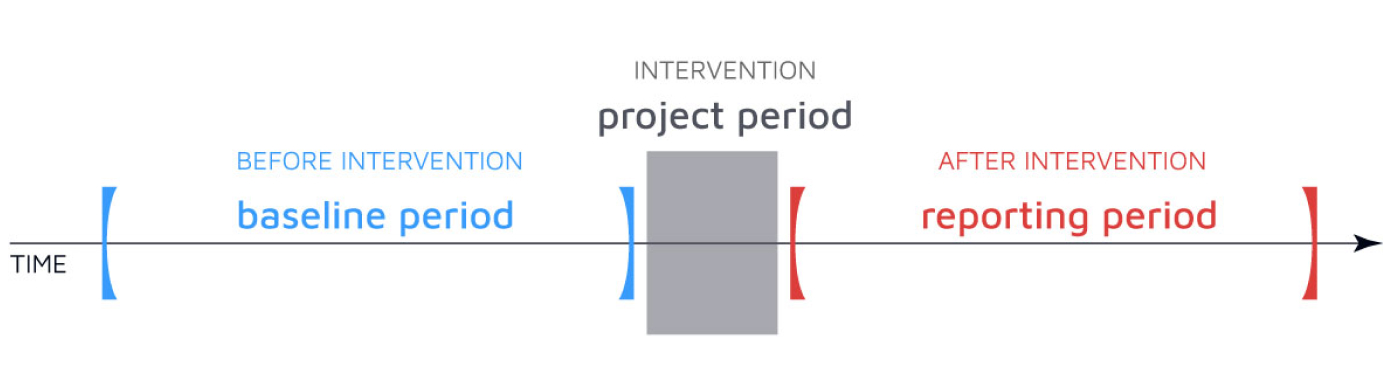Baseline and reporting periods are defined by the endpoints of the project period.

The CalTRACK hourly model defines a building's energy use as the interaction between the building’s temperature dependence, the occupancy status and the time of week.

Before fitting a CalTRACK hourly model three types of variables must be generated: time-of-week, occupancy, and temperature.

## Segmentation by month

To predict the counterfactual during any time period a baseline model for that calendar month (“month-by-month” models) is used. This implies that there can be up to 12 separate models for a particular building - one for predicting the counterfactual in each calendar month.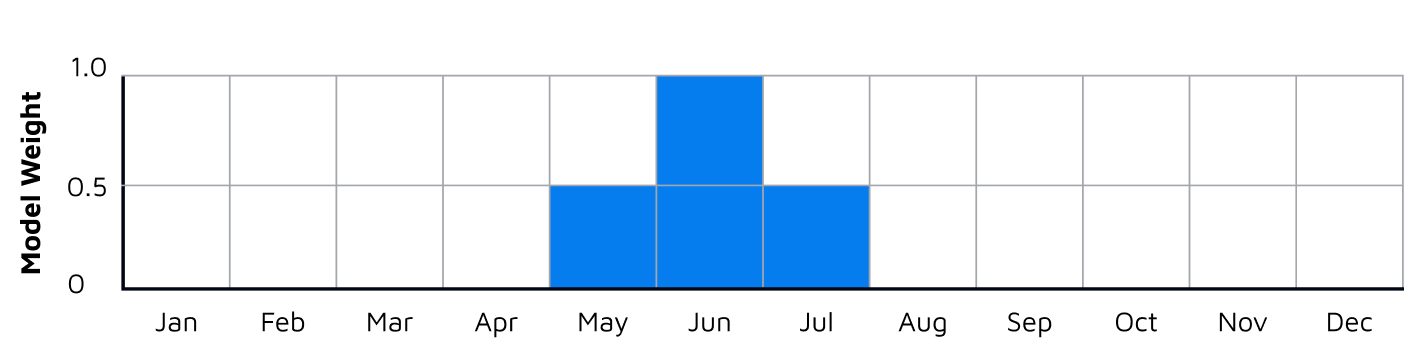Shows model weights for data used to fit the June model, i.e., full weighted June data, half-weighted data from May and July. This same pattern is used for each of the twelve monthly models.

Each model is fit using baseline data comprising (i) data from the same calendar month in the 365 days prior to the intervention date. These data points are given full weight when fitting the model, (ii) data from the previous and subsequent calendar months in the 365 days prior to the intervention date. These data points are given a weight of 0.5 when fitting the model. For example, for a project installed in March 2018, predicting the counterfactual in Jun 2018 will be done using a model fit to baseline data from May, June and July 2017, with weights of 0.5, 1 and 0.5 assigned to the data points in those three months.

## Select hour of week

A week is divided into 168 hourly time-of-week intervals starting on Monday. For example, interval 1 is from midnight to 1 a.m. on Monday morning, interval 2 is from 1 a.m.-2 a.m. and so on. Dummy variables are included in the model for each time of week.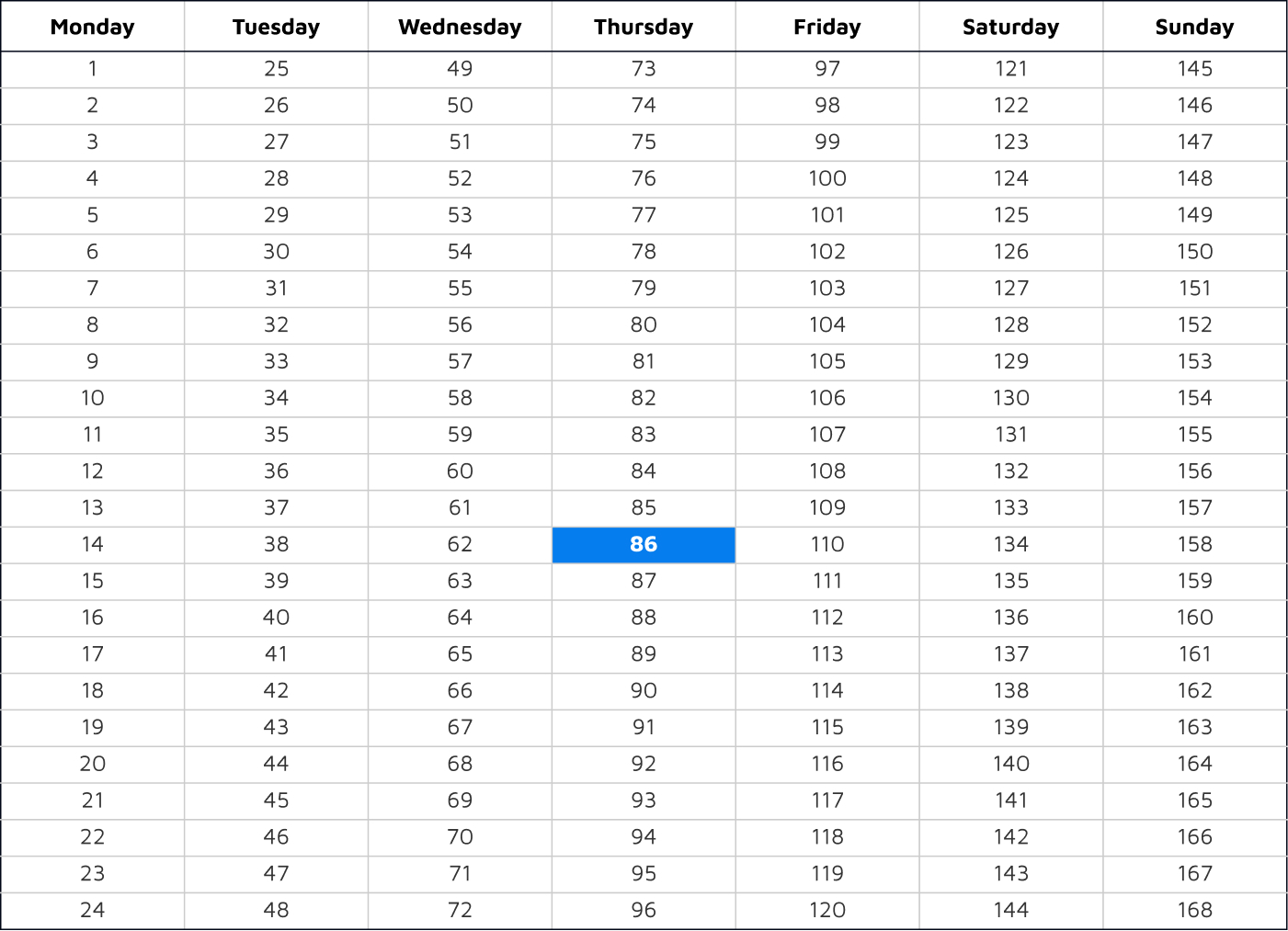A label is applied to each hour of the week, with 1 = mon 00:00-01:00, 168 = sun 23:00-00:00

## Figure out occupancy

The sensitivity of building energy use to temperature may vary depending on the “occupancy” status. This is handled in the Time-Of-Week and Temperature model (TOWT) by segmenting the times-of-week into periods of high load and low load (also referred to as occupied/unoccupied, although the states may not necessarily correspond to occupancy changes).

The segmentation is accomplished using the residuals of a HDD-CDD model that uses fixed balance points. Hours of the week that appear to be in high usage mode most of the time are flagged as “occupied”.

## Bin Temperature

For each temperature data point in the baseline dataset, the outdoor air temperature is used to calculate up to 7 new binned features using an algorithm developed for the original Time-Of-Week and Temperature model (TOWT). This algorithm apportions the temperature into different bins with preselected endpoints as shown in the figure below.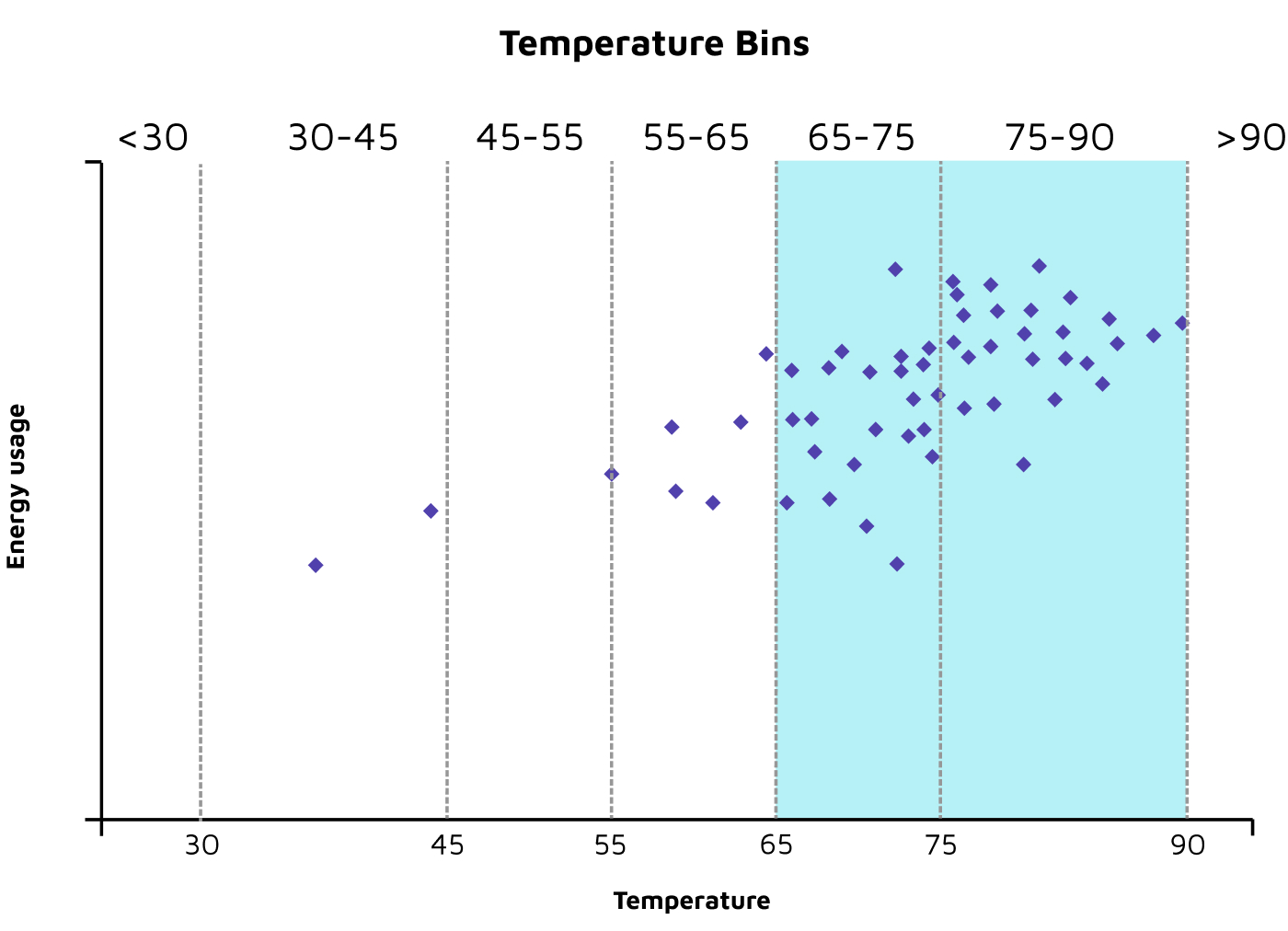Shows the preselected bins each observed temperature is assigned to. Highlighted in blue are the bins meeting data sufficiency criteria. Bins not meeting data sufficiency criteria are combined with the nearest bin meeting data sufficiency requirements.

## Figure out temperature features

Convert each observed temperature for a given hour into a feature by bin to use as input to regression model.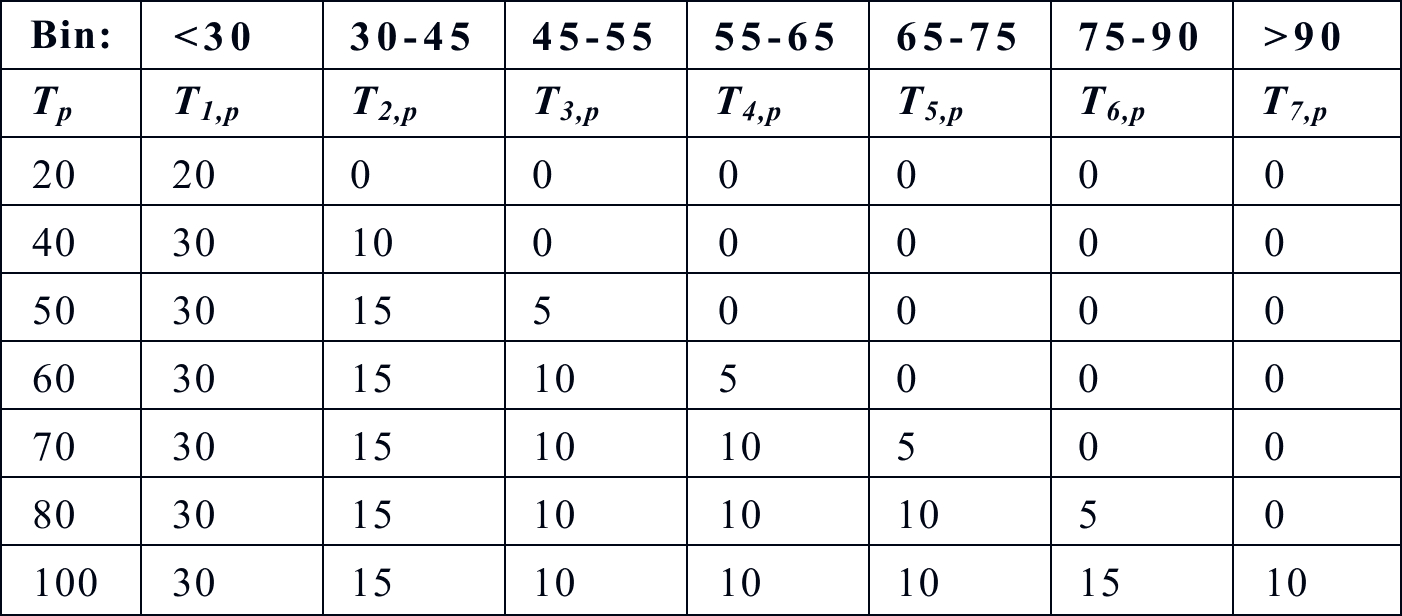Each row of the table above shows an example temperature Tp and 7 computed temperature features Tn,p for the default set of 7 bins.

## Find coefficients

Fit a linear regression model to find the coefficients with the least error.

## Use model to predict energy consumption

Each monthly model has 168 hour-of-week feature coefficients, 1 to 7 temperature bin feature coefficients for the occupied mode, and 1 to 7 temperature bin feature coefficients for the unoccupied mode. To make a model prediction, each feature is multiplied by its respective fitted coefficients and all products are summed to create a final prediction of energy consumption.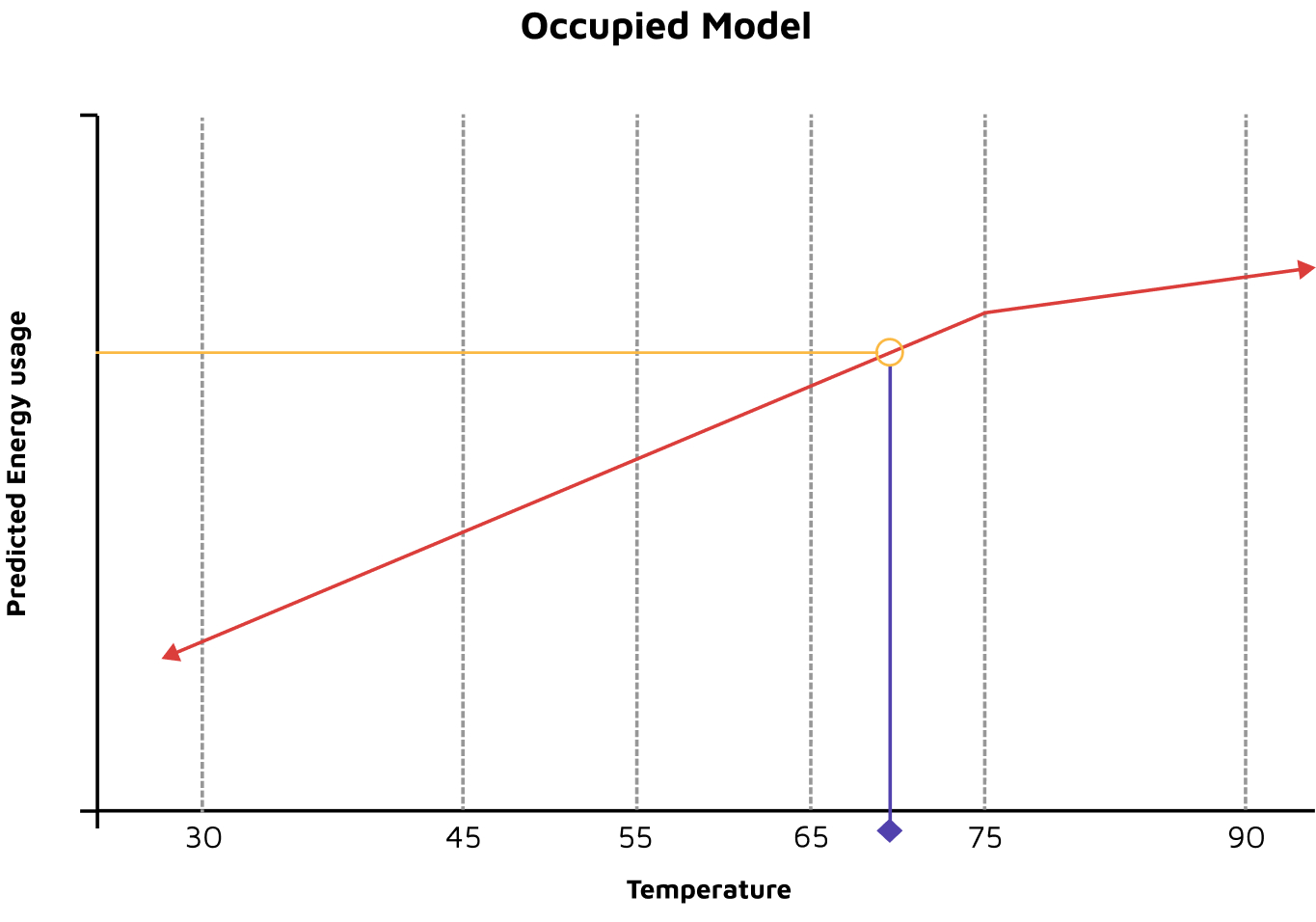Shows using model coefficients to predict the energy consumption temperature-dependent occupied mode component of the final model.

You can learn about CalTRACK Billing & Methods here.